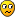## Kakuro Template Generation

For fans of Kakuro

### Kakuro Template Generation

Goodness me, 3 months without a new Kakuro post!I got an email from surendra.jain, asking what method I use to generate Kakuro templates (ie: the blank grid patterns). So I thought it might be of general interest (although given the lack of postings since I was last here suggests I might have an audience of only one!).

The key to a reliable method of template generation (so it seems to me) is to start with the edges and work in!

Assuming we wish a conventional grid (ie: symmetric about the diagonal), then for any size we start with the top and left edges and with reflection this gives us our complete outer edge.

I generated all possible valid edge patterns for various sizes, and selected one at random, but any method will do. Say we started with this simple case (X's represent white cells, dots represent black, - indicates to be decided). I've used an 8x9 grid size but this is completely arbitrary (although it's easier with an odd number of rows, as we will see):

Code: Select all
`X X . X X X . .X - - - - - - .X - - - - - - X. - - - - - - XX - - - - - - X         X - - - - - - .X - - - - - - X. - - - - - - X. . X X X . X X`

Now many cells in rows 2 and 8, and cols 2 and 8, are forced - if there is an X on the outer edge, it's neighbour must also be X, or we'd have orphans (cells must have at least one horizontal and one vertical neighbour):
Code: Select all
`X X . X X X . .X X - X X X - .X X - - - - X X. - - - - - X XX X - - - - X X         X X - - - - - .X X - - - - X X. - X X X - X X. . X X X . X X`

I chose an odd number for the rows because that means the center row (5) must be symmetric. So we only have to decide on a symmetric pattern to complete that row:
Code: Select all
`X X . X X X . .X X - X X X - .X X - - - - X X. - - - - - X XX X . X X . X X         X X - - - - - .X - - - - - X X. - X X X - X X. . X X X . X X`

We have only two more rows to complete - 3 and 4, which reflect to 6 and 7. At this stage I process unassigned cells in row 2, then row 3 until I hit the center and thus complete the grid, but always filling in from the outside in.

For any free cell we check whether it needs to be forced, if not randomly choose between X and .

A forced move means that we might otherwise fail one of these checks:

• maintaining contiguity (prevent disconnection of white cells)

• maintaining the desired maximum run length

If the process gets stuck, we simply start again from the outer edges, or restart the whole process:

Code: Select all
`X X . X X X . .X X . X X X X .X X X X . X X X. . X X X . X XX X . X X . X X         X X . X X X . .X X X . X X X X. X X X X . X X. . X X X . X X`Mathimagics
2017 Supporter

Posts: 1762
Joined: 27 May 2015
Location: Canberra

### Re: Kakuro Template Generation

Thanks for that. It reminds me I haven't created on in a while.

As I'm normally a bit lazy when creating Kakuro puzzles I normally use JSudoku and do not allow repeats in rows or columns. But now I have worked out how to remove row and column constraints I'll try some standard ones.
HATMAN

Posts: 272
Joined: 25 February 2006
Location: Nigeria

### Re: Kakuro Template Generation

Can anyone suggest me a good method to check for multiple solutions in a kakuro puzzle. Also can somebody give me some kakuro puzzles that have multiple soluitons.
surendra.jain

Posts: 27
Joined: 15 August 2015
Location: India

### Re: Kakuro Template Generation

For obtaining puzzles with multiple solutions, one easy way is take a known unique solution, choose a cell at random, add or subtract 1 from both of the corresponding H/V sums, that will generally produce multiple solution cases.

Not sure what is meant by the other question - as with all puzzle types, one normally applies a solver with an optional setting to either count all solutions, or to stop when a 2nd solution is found (uniqueness testing).

If you are testing puzzles where you start with a particular solution, then test the corresponding puzzle (generating the sums from the grid content), then there are some cases where multiple solutions can be predicted. See my thread on Kakuro Solution Uniqueness for examples of cycles (sum-preserving).Mathimagics
2017 Supporter

Posts: 1762
Joined: 27 May 2015
Location: Canberra